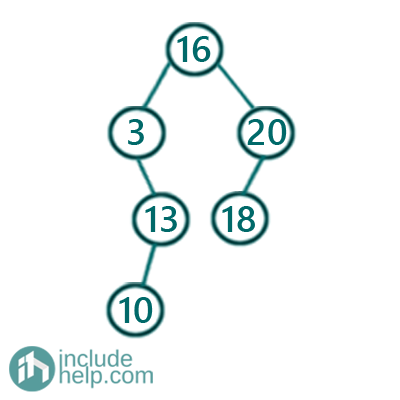# Check if the given array can represent inorder traversal of a BST

In this article, we are going to see how to check if the given array can represent inorder traversal of a BST?
Submitted by Radib Kar, on November 01, 2020

In this article, we are going to see how to find whether a given array can represent inorder traversal of a BST or not. As we already know that inorder traversal of a Binary Search Tree produces a sorted list which is strictly increasing. So if an array can represent inorder traversal for a BST, then it has to be strictly increasing. So, we just need to check whether the array is strictly increasing or not.

For example,

[3, 10, 13, 16, 18, 20]

Is a strictly increasing array and thus it can represent inorder traversal of a Binary search tree. Below is the create BST.But if the given array is [3, 2] or if it's [2, 2, 4] then it's not a valid representation of BST inorder traversal.

Below is the C++ implementation:

```#include <bits/stdc++.h>
using namespace std;

//function which returns true if it's a valid inorder
//traversal for a BST, else return false
int isInorderTraversal(vector<int> a, int n)
{
//base case, a NULL tree or a single node is always a BST
if (n == 0 || n == 1)
return true;
//check if it's a sorted list(strictly increasing only)
for (int i = 0; i < n - 1; i++) {
if (a[i] >= a[i + 1])
return false;
}

return true;
}

int main()
{
int t, n, item;

cout << "Enter number of elements in the list" << endl;
cin >> n;

cout << "Enter the elements" << endl;

vector<int> arr(n);
for (int i = 0; i < n; i++) {
cin >> arr[i];
}

bool result = isInorderTraversal(arr, n);

if (result)
cout << "This array is a valid inorder traversal for a BST" << endl;
else
cout << "This array is an invalid inorder traversal for a BST" << endl;

return 0;
}
```

Output:

```RUN 1:
Enter number of elements in the list
6
Enter the elements
3 10 13 16 18 20
This array is a valid inorder traversal for a BST

RUN 2:
Enter number of elements in the list
2
Enter the elements
3 2
This array is an invalid inorder traversal for a BST
```